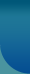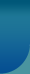Home >> Basic Concepts >> Terms of Sociology - Z

## Terms of Sociology

A   B   C   D   E   F   G   H   I   J   K   L   M
N   O   P   Q   R   S   T   U   V   W   X   Y   Z

Z score
A statistical score expressed in terms of its relative position in the frequency distribution of which it is a part. A score is converted to a z score so that it may be compared with scores from other tests or measuring instruments. A Z score is obtained by subtracting a raw score from the arithmetic mean of the distribution of scores and dividing by the standard deviation of the distribution. The Z score is often referred to as the standard score and occasionally as the sigma score.

Zipf migration hypothesis
An hypothesis in ecology proposed by George K Zipf that states that other factors such as income level, unemployment being equal, the number of persons who migrate between two communities (in both directions) will be equal to the product of the population of the two communities divided by the shortest distance between the communities. The hypothesis is often expressed in terms of the formula P1.P2/D in which P1 is the population of one community ,P2 the population of the other community and D the shortest distance between the two communities. It is sometimes referred to as the P1P2/D hypothesis and also the hypothesis on the intercity movement of persons and the minimum equation hypothesis.© 2023 Sociology Guide.Com